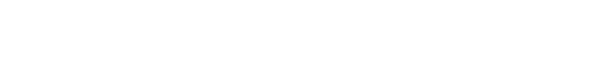# Dart – Understanding Class Initialization Order

I wrote simple code to understand class initialization order. Dart offers late keyword to check null at runtime instead of compile-time. Without its keyword, we need to make the variable datatype nullable. It’s useful when we know the variable can’t be null when it is actually used. Do you already know in which order those classes are initialized?

The following will be compared in this article.

• static final
• late final
• super class

We can define static variables and late keyword but do you already know which is initialized first? Let’s check in this article.

## Which is initialized first, Member or static?

We can define static variable but I was not sure when static variable is initialized. Is it initialized when the package is loaded or when it is actually used? Following is the test code.

// mart.dart
void main() {
var instance = MyStatic(1);
instance.execute();
}

// Static.dart
class _Normal {
final int num;
_Normal(this.num) {
print("Normal($num)"); } @override String toString() { return "instance of _Normal($num)";
}
}

class MyStatic {
static final instance1 = _Normal(1);
final instance2 = _Normal(2);
MyStatic(int num) {
print("MyStatic($num)"); } execute() { print("--- execute start ---"); print(instance1.toString()); print(instance2.toString()); print("--- execute end ---"); } } Do you know in which order those classes are initialized? Let’s check the result. $ dart ./src/main.dart
Normal(2)
MyStatic(1)
--- execute start ---
Normal(1)
instance of _Normal(1)
instance of _Normal(2)
--- execute end ---

A member variable instance2 is first initialized. After that, MyStatic constructor is called. Static variable is initialized neither when its package is loaded nor when the class is initialized. It is initialized when the variable is actually used. It’s used in execute function so Normal(1) is written after --- execute start ---.

## When is a variable initialized when using late keyword

The next thing to check is late keyword. When is it initialized with late keyword? I added some lines.

class MyStatic {
static final instance1 = _Normal(1);
final instance2 = _Normal(2);
late final _Normal instance3; // added
late final _Normal instance4 = _Normal(4); // added
MyStatic(int num) {
print("MyStatic($num)"); instance3 = _Normal(3); // added } execute() { print("--- execute start ---"); print(instance1.toString()); print(instance2.toString()); print(instance3.toString()); // added print(instance4.toString()); // added print("--- execute end ---"); } } We don’t have to assign anything to instance3 on the same line because of late keyword. Its data type must be nullable without late keyword. It means that we need to append a question mark at the end like the following. final _Normal? instance3; If the variable definitely has value when it’s used, we can specify late keyword and we don’t have to check whether it’s null or not. Is instance4 initialized when it is actually used because of late keyword? Let’s check the result. $ dart ./src/main.dart
Normal(2)
MyStatic(1)
Normal(3)
--- execute start ---
Normal(1)
instance of _Normal(1)
instance of _Normal(2)
instance of _Normal(3)
Normal(4)
instance of _Normal(4)
--- execute end ---

Yes, the result was the same as what we expected. The instance with late keyword is initialized when it is actually used. It’s the same timing as static variable.

## Initialization order when a class extends a subclass

The next step is extended class. I created the following class.

class ExtendedMyStatic extends MyStatic {
final _Normal normal90;
final _Normal normal91 = _Normal(91);
late final _in constructorNormal normal92;
ExtendedMyStatic(int num)
: normal90 = _Normal(90),
super(num) {
print("ExtendedMyStatic($num)"); normal92 = _Normal(92); } } ExtendedMyStatic class must call super class constructor because subclass constructor requires an argument. That’s why super(num) is there. There is initialization in between. This initialization step is called before calling super class constructor. Let’s see the result. $ dart ./src/main.dart
Normal(91)  # initialized at the same line as declaration
Normal(90)  # initialized in constructor
Normal(2)   # final in subclass
MyStatic(1) # constructor of subclass
Normal(3)   # initialized in constructor in subclass
ExtendedMyStatic(1)
Normal(92)  # late final in extended class
--- execute start ---
Normal(1)   # static final in subclass
instance of _Normal(1)
instance of _Normal(2)
instance of _Normal(3)
Normal(4)   # late final in subclass
instance of _Normal(4)
--- execute end ---

The initialization order is following.

1. member variable
2. initialization step defined between constructor function and super constructor call
3. super constructor
4. repeat 1 – 3 steps in the super class
5. self constructor

late final and static variables are initialized when they are first accessed.

## End

I haven’t checked the official page but it explains the initialization order. However, it is always good to try it yourself.

You can clone my repository if you want.

GitHub - yuto-yuto/blogpost-dart
Contribute to yuto-yuto/blogpost-dart development by creating an account on GitHub.